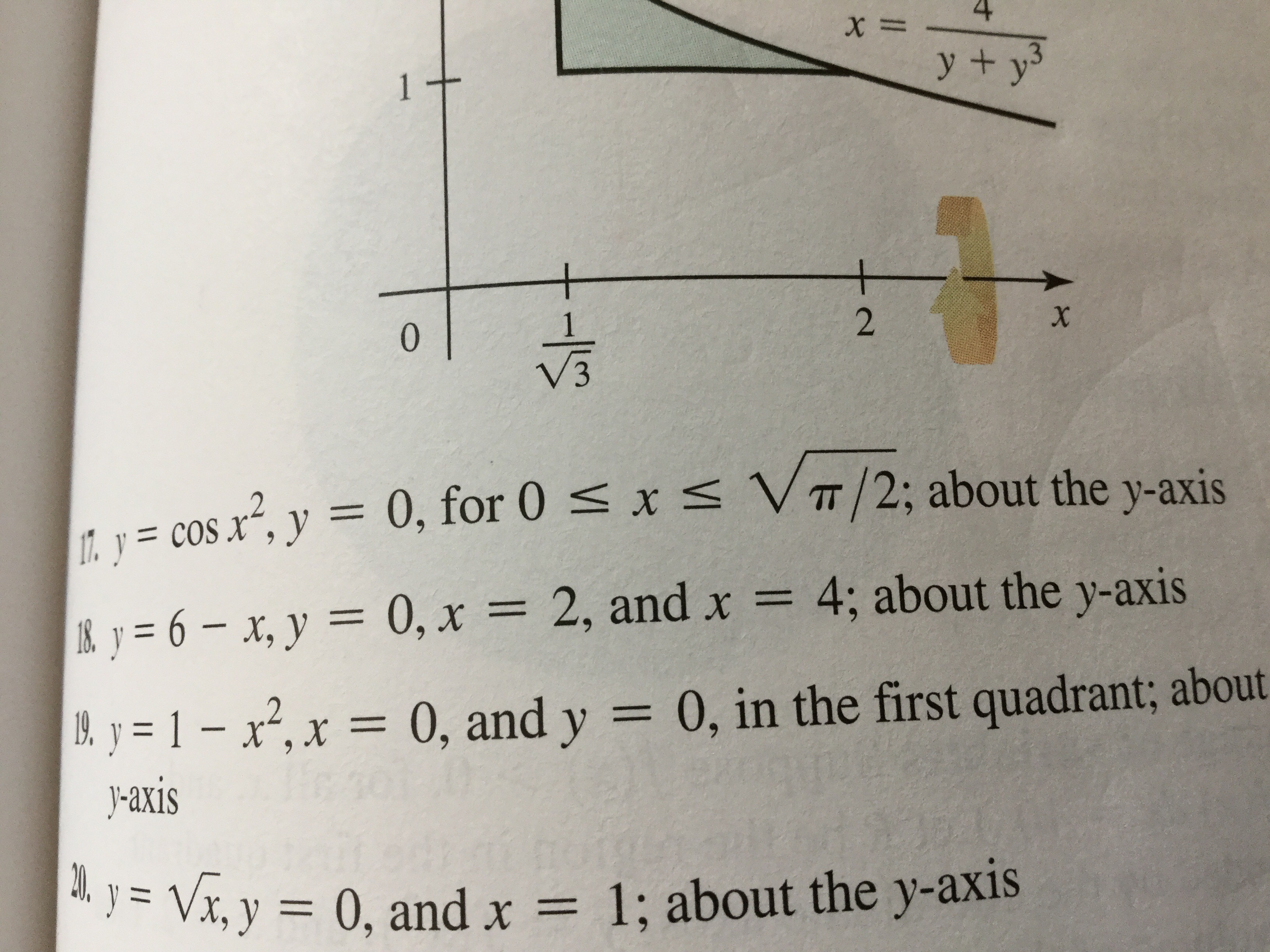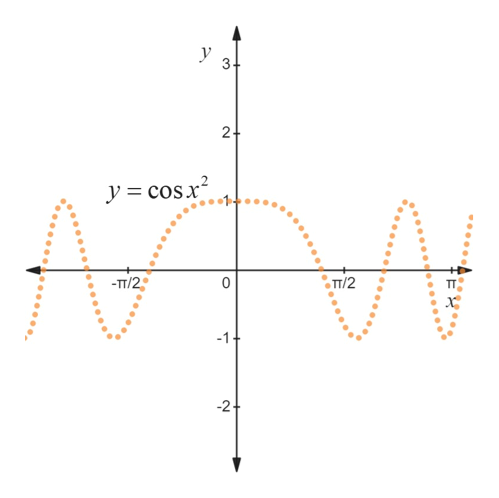X=y + y1+2X01. y =cos x, y 0, for 0 x VIT/2; about the y-axis&y=6-x, y = 0, x = 2, and x = 4; about the y-axis0, in the first quadrant; aboutB. y=1-x2, x = 0, and y-axisVx, y 0, and x = 1; about the y-axis

Question

I am confused on #17. Not really sure how to sketch it.help_outlineImage TranscriptioncloseX= y + y 1+ 2 X 0 1. y =cos x, y 0, for 0 x VIT/2; about the y-axis &y=6-x, y = 0, x = 2, and x = 4; about the y-axis 0, in the first quadrant; about B. y=1-x2, x = 0, and y -axis Vx, y 0, and x = 1; about the y-axis fullscreen
Step 1

The curve y=cos x2 is sketche...help_outlineImage Transcriptionclosey 3 2+ y =cos2 -TT/2 TT/2 -1 2 fullscreen

Want to see the full answer?

See Solution

Want to see this answer and more?

Our solutions are written by experts, many with advanced degrees, and available 24/7

See Solution
Tagged in

Calculus